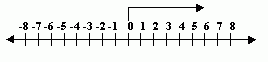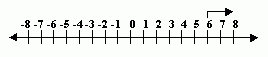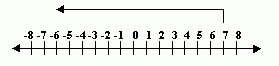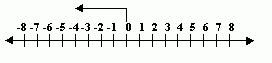Name: ___________________Date:___________________

 Email us to get an instant 20% discount on highly effective K-12 Math & English kwizNET Programs!

 Adding two positive numbers: The operation is addition and the result is a positive number. Adding one positive and one negative number: The operation is subtraction and the result can be either positive or negative. Do not confuse the sign of the integer with the operation being performed. Adding two negative numbers: The operation is addition and the result is a negative number. Examples:Directions: Answer the following questions. Also write at least ten examples of your own.Name: ___________________Date:___________________

 Q 1: 0 + 6 =68-6 Q 2: 6 + 2 =7-88 Q 3: 7 + -13 = 7 - 13 =-676 Q 4: 0 + -4 = 0 - 4 =-440 Question 5: This question is available to subscribers only! Question 6: This question is available to subscribers only!